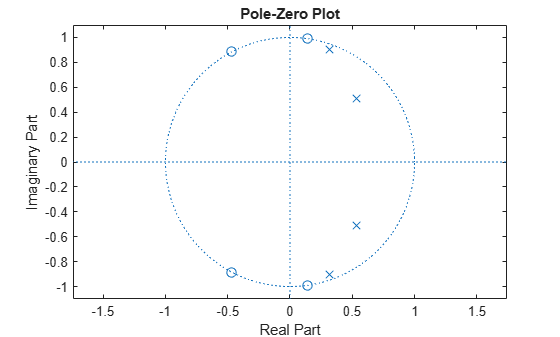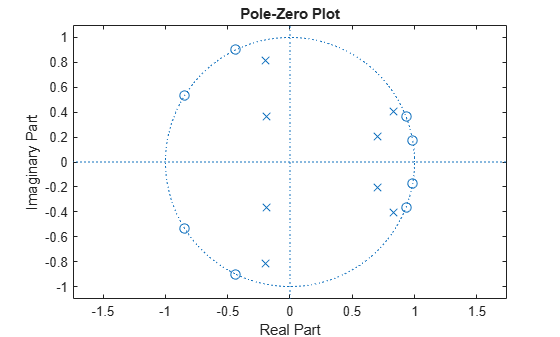# zplane

Zero-pole plot for discrete-time systems

## Syntax

``zplane(z,p)``
``zplane(b,a)``
``[hz,hp,ht] = zplane(___)``
``zplane(d)``
``[vz,vp,vk] = zplane(d)``

## Description

example

````zplane(z,p)` plots the zeros specified in column vector `z` and the poles specified in column vector `p` in the current figure window. The symbol `'o'` represents a zero and the symbol `'x'` represents a pole. The plot includes the unit circle for reference. If `z` and `p` are matrices, then `zplane` plots the poles and zeros in the columns of `z` and `p` in different colors.```

example

````zplane(b,a)`, where `b` and `a` are row vectors, first uses `roots` to find the zeros and poles of the transfer function represented by the numerator coefficients `b` and the denominator coefficients `a`.```
````[hz,hp,ht] = zplane(___)` returns vectors of handles to the zero lines, `hz`, and the pole lines, `hp`. `ht` is a vector of handles to the axes/unit circle line and to text objects, which are present when there are multiple zeros or poles.```
````zplane(d)` finds the zeros and poles of the transfer function represented by the digital filter, `d`. Use `designfilt` to generate `d` based on frequency-response specifications. The pole-zero plot is displayed in FVTool.```
````[vz,vp,vk] = zplane(d)` returns the zeros (vector `vz`), poles (vector `vp`), and gain (scalar `vk`) corresponding to the digital filter `d`.```

## Examples

collapse all

For data sampled at 1000 Hz, plot the poles and zeros of a 4th-order elliptic lowpass digital filter with cutoff frequency 200 Hz, 3 dB of ripple in the passband, and 30 dB of attenuation in the stopband.

```[z,p,k] = ellip(4,3,30,200/500); zplane(z,p) grid title('4th-Order Elliptic Lowpass Digital Filter')```Create the same filter using `designfilt`. Use `zplane` to plot the poles and zeros. Note that this syntax of `zplane` calls `fvtool`.

```d = designfilt('lowpassiir','FilterOrder',4,'PassbandFrequency',200, ... 'PassbandRipple',3,'StopbandAttenuation',30, ... 'DesignMethod','ellip','SampleRate',1000); zplane(d)```Design an 8th-order Chebyshev Type II bandpass filter with a stopband attenuation of 20 dB. Specify the stopband edge frequencies as $\pi /8$ rad/sample and $5\pi /8$ rad/sample.

`[b,a] = cheby2(8/2,20,[1 5]/8);`

Use `zplane` to plot the poles and zeros of the transfer function.

`zplane(b,a)`Visualize the zero-phase response of the filter. Overlay the unit circle and the pole and zero locations.

```[hw,fw] = zerophase(b,a,1024,"whole"); z = roots(b); p = roots(a); plot3(cos(fw),sin(fw),hw) hold on plot3(cos(fw),sin(fw),zeros(size(fw)),'--') plot3(real(z),imag(z),zeros(size(z)),'o') plot3(real(p),imag(p),zeros(size(p)),'x') hold off xlabel("Real") ylabel("Imaginary") view(35,40) grid```## Input Arguments

collapse all

Zeros and poles, specified as column vectors or matrices. If `z` and `p` are matrices, then `zplane` plots the poles and zeros in the columns of `z` and `p` in different colors.

Data Types: `single` | `double`
Complex Number Support: Yes

Transfer function coefficients, specified as row vectors. The transfer function is defined in terms of z–1:

`$H\left(z\right)=\frac{B\left(z\right)}{A\left(z\right)}=\frac{b\left(1\right)+b\left(2\right){z}^{-1}+\cdots +b\left(n+1\right){z}^{-n}}{a\left(1\right)+a\left(2\right){z}^{-1}+\cdots +a\left(m+1\right){z}^{-m}}$`

Example: `b = [1 3 3 1]/6` and ```a = [3 0 1 0]/3``` specify a third-order Butterworth filter with normalized 3-dB frequency 0.5π rad/sample.

Data Types: `single` | `double`
Complex Number Support: Yes

Digital filter, specified as a `digitalFilter` object. Use `designfilt` to generate a digital filter based on frequency-response specifications.

Example: ```d = designfilt('lowpassiir','FilterOrder',3,'HalfPowerFrequency',0.5)``` specifies a third-order Butterworth filter with normalized 3-dB frequency 0.5π rad/sample.

## Output Arguments

collapse all

Vectors of handles to the zero lines, `hz`, and the pole lines, `hp`, of the pole-zero plot. `ht` is a vector of handles to the axes/unit circle line and to text objects, which are present when there are multiple zeros or poles. If there are no zeros or no poles, `hz` or `hp` is the empty matrix, `[]`.

Zeros, poles, and gain of a digital filter, `d`, returned as column vectors and a scalar.

## Tips

• You can override the automatic scaling of `zplane` using

`axis([xmin xmax ymin ymax])`

after calling `zplane`. This scaling is useful when one or more zeros or poles have such a large magnitude that the others are grouped tightly around the origin and are hard to distinguish.